# Weyl group

(diff) ← Older revision | Latest revision (diff) | Newer revision → (diff)

The Weyl group of symmetries of a root system. Depending on the actual realization of the root system, different Weyl groups are considered: Weyl groups of a semi-simple splittable Lie algebra, of a symmetric space, of an algebraic group, etc.

Letbe a connected affine algebraic group defined over an algebraically closed field. The Weyl group ofwith respect to a torusis the quotient groupconsidered as a group of automorphisms ofinduced by the conjugations ofby elements of. Here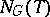is the normalizer (cf. Normalizer of a subset) and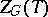is the centralizer ofin. The group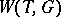is finite. Ifis a maximal torus,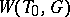is said to be the Weyl group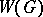of the algebraic group. This definition does not depend on the choice of a maximal torus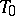(up to isomorphism). The action by conjugation of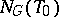on the set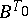of Borel subgroups (cf. Borel subgroup) incontaininginduces a simply transitive action ofon. The action by conjugation ofoninduces an adjoint action ofon the Lie algebraof. Letbe the set of non-zero weights of the weight decomposition ofwith respect to this action, which means thatis the root system ofwith respect to(cf. Weight of a representation of a Lie algebra).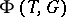is a subset of the group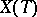of rational characters of the torus, andis invariant with respect to the action ofon.

Letbe a reductive group, let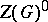be the connected component of the identity of its centre and letbe a maximal torus of. The vector space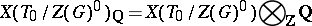is canonically identified with a subspace of the vector space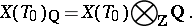As a subset of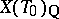, the set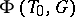is a reduced root system in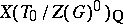, and the natural action ofondefines an isomorphism betweenand the Weyl group of the root system. Thus,displays all the properties of a Weyl group of a reduced root system; e.g. it is generated by reflections (cf. Reflection).

The Weyl group of a Tits system is a generalization of this situation (for its exact definition see Tits system).

The Weyl groupof a finite-dimensional reductive Lie algebraover an algebraically closed field of characteristic zero is defined as the Weyl group of its adjoint group. The adjoint action ofin the Cartan subalgebra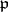ofis a faithful representation of. The groupis often identified with the image of this representation, being regarded as the corresponding linear group ingenerated by the reflections. The concept of a "Weyl group" was first used by H. Weyl

in the special case of finite-dimensional semi-simple Lie algebras over the field of complex numbers. A Weyl group may also be defined for an arbitrary splittable semi-simple finite-dimensional Lie algebra, as the Weyl group of its root system. A relative Weyl group may be defined for an affine algebraic groupdefined over an algebraically non-closed field. Ifis a maximal-split torus of, then the quotient group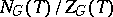(the normalizer ofover its centralizer in), regarded as the group of automorphisms ofinduced by the conjugations ofby elements of, is said to be the relative Weyl group of.

For the Weyl group of a symmetric space, see Symmetric space. The Weyl group of a real connected non-compact semi-simple algebraic group is identical with the Weyl group of the corresponding symmetric space. For the affine Weyl group see Root system.

How to Cite This Entry:
Weyl group. Encyclopedia of Mathematics. URL: http://encyclopediaofmath.org/index.php?title=Weyl_group&oldid=18086
This article was adapted from an original article by V.L. Popov (originator), which appeared in Encyclopedia of Mathematics - ISBN 1402006098. See original article